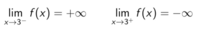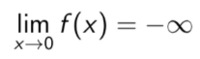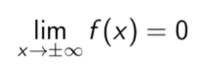Another Another simple limits question (graphing this time)

Wahamuka

New member
This is in our lesson infinite limits and limits at infinity

Question and my answer attached in the file.

I have no way of checking my answer (can desmos solve this? Idk how)

Attachments

• 59.1 KB Views: 14
• 1.7 MB Views: 14

HallsofIvy

Elite Member
You are told that f is a function. So your graph for x< 0 cannot be right.

•Harry_the_cat

Wahamuka

New member
You are told that f is a function. So your graph for x< 0 cannot be right.
Didnt notice that, but if thats the case how do I express lim x -> +- inf f(x) = 0. Specifically the +- inf

Harry_the_cat

Senior Member
Let me step you through it.

1. f(2) = 0 means (2, 0) is on the graph. Good you've got that.2. Notice that moving from the left towards 3,the graph gets infinitely high.
Moving from the right towards 3, the graph gets infinitely low.
This suggests that there might be an asymptote at x=3.
So you've drawn the graph correctly near x=3. Good.

3.This means that coming in to 0 from the left AND the right the graph goes down to negative infinity.
So to the left of 0 you have it correct but I see you have crossed out the bit coming in from the right!

4.This means that at the far right of your graph and the far left of your graph the graph approaches the x-axis.
On the right you are correct. And on the left below the x-axis you are correct.

Now get rid of the bit above the x-axis on the left. That's incorrect (as Halls said it is a function after all).

Now for the bit between 0 and 3 Your arrow at the bottom left of this section is correct (the one you crossed out!).
The arrow at the top right of this section is also correct as is the point (2, 0). So now join the bottom arrow through the point (2, 0) to the top arrow.
There will be some sort of inflection point along the way but you are not given enough info to locate it. (That's why the question says graph a function not graph the function.)

Wahamuka

New member
Let me step you through it.

1. f(2) = 0 means (2, 0) is on the graph. Good you've got that.

View attachment 12890
2. Notice that moving from the left towards 3,the graph gets infinitely high.
Moving from the right towards 3, the graph gets infinitely low.
This suggests that there might be an asymptote at x=3.
So you've drawn the graph correctly near x=3. Good.

3.View attachment 12891
This means that coming in to 0 from the left AND the right the graph goes down to negative infinity.
So to the left of 0 you have it correct but I see you have crossed out the bit coming in from the right!

4. View attachment 12892
This means that at the far right of your graph and the far left of your graph the graph approaches the x-axis.
On the right you are correct. And on the left below the x-axis you are correct.

Now get rid of the bit above the x-axis on the left. That's incorrect (as Halls said it is a function after all).

Now for the bit between 0 and 3 Your arrow at the bottom left of this section is correct (the one you crossed out!).
The arrow at the top right of this section is also correct as is the point (2, 0). So now join the bottom arrow through the point (2, 0) to the top arrow.
There will be some sort of inflection point along the way but you are not given enough info to locate it. (That's why the question says graph a function not graph the function.)
This means that at the far right of your graph and the far left of your graph the graph approaches the x-axis.
“ON THE RIGHT YOU ARE CORRECT”. And on the left below the x-axis you are correct.

But I didn’t draw anything on the right going to infinity... so I’m wrong there right?

This is my new graph, is it correct?
Thanks so much for your long post by the way very helpful

Attachments

• 1.9 MB Views: 6

Harry_the_cat

Senior Member
On the right of the graph ie as x -> infinity the graph approaches the x-axis not infinity. It's approaching the x-axis from below.
I see I missed the open dot on the right of your original graph. Get rid of that dot (why is it there?) and put an arrow.
(The extra bit you've added on can be deleted - although actually your latest graph does fit all the conditions plus more. But it does answer the question correctly!)

•Wahamuka

Wahamuka

New member
On the right of the graph ie as x -> infinity the graph approaches the x-axis not infinity. It's approaching the x-axis from below.
I see I missed the open dot on the right of your original graph. Get rid of that dot (why is it there?) and put an arrow.
(The extra bit you've added on can be deleted - although actually your latest graph does fit all the conditions plus more. But it does answer the question correctly!)
Thanks so much its all clear now!

Jomo

Elite Member
As The Cat has said your graph has more which is fine. No problem at all--unless you think that the more has to be there. You do know that there did NOT have to be a vertical asymptote to the right of x=3 (at around x=4 or x=5on your graph)?

Wahamuka

New member
As The Cat has said your graph has more which is fine. No problem at all--unless you think that the more has to be there. You do know that there did NOT have to be a vertical asymptote to the right of x=3 (at around x=4 or x=5on your graph)?
That makes sense, ill remove that and instead put the arrow going to the right infinity at the bottom with the other arrow pointing down